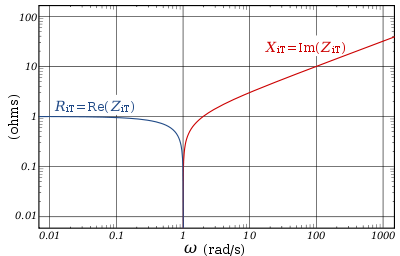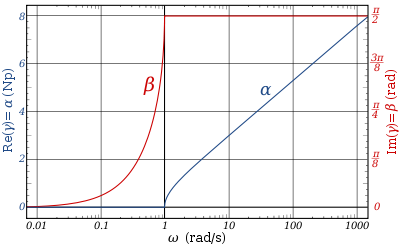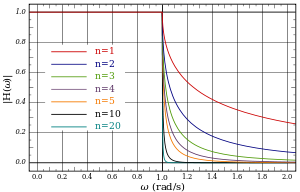# Constant k filter

﻿
Constant k filter
Linear analog electronic filters
edit

Constant k filters, also k-type filters, are a type of electronic filter designed using the image method. They are the original and simplest filters produced by this methodology and consist of a ladder network of identical sections of passive components. Historically, they are the first filters that could approach the ideal filter frequency response to within any prescribed limit with the addition of a sufficient number of sections. However, they are rarely considered for a modern design, the principles behind them having been superseded by other methodologies which are more accurate in their prediction of filter response.

## History

Constant k filters were invented by George Campbell. He published his work in 1922, but had clearly invented the filters some time before, as his colleague at AT&T Co, Otto Zobel, was already making improvements to the design at this time. Campbell's filters were far superior to the simpler single element circuits that had been used previously. Campbell called his filters electric wave filters, but this term later came to mean any filter that passes waves of some frequencies but not others. Many new forms of wave filter were subsequently invented; an early (and important) variation was the m-derived filter by Zobel who coined the term constant k for the Campbell filter in order to distinguish them.

The great advantage Campbell's filters had over the RL circuit and other simple filters of the time was that they could be designed for any desired degree of stop band rejection or steepness of transition between pass band and stop band. It was only necessary to add more filter sections until the desired response was obtained.

The filters were designed by Campbell for the purpose of separating multiplexed telephone channels on transmission lines, but their subsequent use has been much more widespread than that. The design techniques used by Campbell have largely been superseded. However, the ladder topology used by Campbell with the constant k is still in use today with implementations of modern filter designs such as the Tchebyscheff filter. Campbell gave constant k designs for low-pass, high-pass and band-pass filters. Band-stop and multiple band filters are also possible.

## Terminology

Some of the impedance terms and section terms used in this article are pictured in the diagram below. Image theory defines quantities in terms of an infinite cascade of two-port sections, and in the case of the filters being discussed, an infinite ladder network of L-sections. Here "L" should not be confused with the inductance L – in electronic filter topology, "L" refers to the specific filter shape which resembles inverted letter "L".

The sections of the hypothetical infinite filter are made of series elements having impedance 2Z and shunt elements with admittance 2Y. The factor of two is introduced for mathematical convenience, since it is usual to work in terms of half-sections where it disappears. The image impedance of the input and output port of a section will generally not be the same. However, for a mid-series section (that is, a section from halfway through a series element to halfway through the next series element) will have the same image impedance on both ports due to symmetry. This image impedance is designated ZiT due to the "T" topology of a mid-series section. Likewise, the image impedance of a mid-shunt section is designated ZiΠ due to the "Π" topology. Half of such a "T" or "Π" section is called a half-section, which is also an L-section but with half the element values of the full L-section. The image impedance of the half-section is dissimilar on the input and output ports: on the side presenting the series element it is equal to the mid-series ZiT, but on the side presenting the shunt element it is equal to the mid-shunt ZiΠ . There are thus two variant ways of using a half-section.

Parts of this article or section rely on the reader's knowledge of the complex impedance representation of capacitors and inductors and on knowledge of the frequency domain representation of signals.

## DerivationConstant k low-pass filter half section. Here inductance L is equal Ck2Image impedance ZiT of a constant k prototype low-pass filter is plotted vs. frequency ω. The impedance is purely resistive (real) below ωc, and purely reactive (imaginary) above ωc.

The building block of constant k filters is the half-section "L" network, composed of a series impedance Z, and a shunt admittance Y. The "k" in "constant k" is the value given by,$k^2=\frac{Z}{Y}$

Thus, k will have units of impedance, that is, ohms. It is readily apparent that in order for k to be constant, Y must be the dual impedance of Z. A physical interpretation of k can be given by observing that k is the limiting value of Zi as the size of the section (in terms of values of its components, such as inductances, capacitances, etc.) approaches zero, while keeping k at its initial value. Thus, k is the characteristic impedance, Z0, of the transmission line that would be formed by these infinitesimally small sections. It is also the image impedance of the section at resonance, in the case of band-pass filters, or at ω = 0 in the case of low-pass filters. For example, the pictured low-pass half-section has$k = \sqrt{\frac{i\omega L}{i \omega C}} = \sqrt{\frac{L}{C}}$.

Elements L and C can be made arbitrarily small while retaining the same value of k. Z and Y however, are both approaching zero, and from the formulae (below) for image impedances,$\lim_{Z,Y \to 0}Z_\mathrm i=k$.

### Image impedance

The image impedances of the section are given by${Z_\mathrm{iT}}^2=Z^2 + k^2$

and$\frac{1}{{Z_\mathrm{i\Pi}}^2}={Y_\mathrm{i\Pi}}^2=Y^2 + \frac{1}{k^2}$

Provided that the filter does not contain any resistive elements, the image impedance in the pass band of the filter is purely real and in the stop band it is purely imaginary. For example, for the pictured low-pass half-section,${Z_\mathrm{iT}}^2=-(\omega L)^2 + \frac{L}{C}$

The transition occurs at a cut-off frequency given by$\omega_c=\frac{1}{\sqrt{LC}}$

Below this frequency, the image impedance is real,$Z_\mathrm{iT}=L\sqrt{\omega_c^2-\omega^2}$

Above the cut-off frequency the image impedance is imaginary,$Z_\mathrm{iT}=iL\sqrt{\omega^2-\omega_c^2}$

### Transmission parametersThe transfer function of a constant k prototype low-pass filter for a single half-section showing attenuation in nepers and phase change in radians.

The transmission parameters for a general constant k half-section are given by$\gamma=\sinh^{-1}\frac{Z}{k}$

and for a chain of n half-sections$\gamma_n=n\gamma\,\!$

For the low-pass L-shape section, below the cut-off frequency, the transmission parameters are given by$\gamma=\alpha+i\beta=0+i\sin^{-1}\frac{\omega}{\omega_c}$

That is, the transmission is lossless in the pass-band with only the phase of the signal changing. Above the cut-off frequency, the transmission parameters are:$\gamma=\alpha+i\beta=\cosh^{-1}\frac{\omega}{\omega_c}+i\frac{\pi}{2}$

### Prototype transformations

The presented plots of image impedance, attenuation and phase change correspond to a low-pass prototype filter section. The prototype has a cut-off frequency of ωc = 1 rad/s and a nominal impedance k = 1 Ω. This is produced by a filter half-section with inductance L = 1 henry and capacitance C = 1 farad. This prototype can be impedance scaled and frequency scaled to the desired values. The low-pass prototype can also be transformed into high-pass, band-pass or band-stop types by application of suitable frequency transformations.Gain response, H(ω) for a chain of n low-pass constant-k filter half-sections.

Several L-shape half-sections may be cascaded to form a composite filter. Like impedance must always face like in these combinations. There are therefore two circuits that can be formed with two identical L-shaped half-sections. Where a port of image impedance ZiT faces another ZiT, the section is called a Π section. Where ZiΠ faces ZiΠ the section so formed is a T section. Further additions of half-sections to either of these section forms a ladder network which may start and end with series or shunt elements.

It should be borne in mind that the characteristics of the filter predicted by the image method are only accurate if the section is terminated with its image impedance. This is usually not true of the sections at either end, which are usually terminated with a fixed resistance. The further the section is from the end of the filter, the more accurate the prediction will become, since the effects of the terminating impedances are masked by the intervening sections.

Image filter sections

 N.B. Textbooks and design drawings usually show the unbalanced implementations, but in telecoms it is often required to convert the design to the balanced implementation when used with balanced lines. edit

Wikimedia Foundation. 2010.

### Look at other dictionaries:

• Filter konstanter absoluter Bandbreite — Filter (n) konstanter absoluter Bandbreite eng constant bandwidth filter …   Arbeitssicherheit und Gesundheitsschutz Glossar

• Filter design — is the process of designing a filter (in the sense in which the term is used in signal processing, statistics, and applied mathematics), often a linear shift invariant filter, which satisfies a set of requirements, some of which are contradictory …   Wikipedia

• Filter konstanter relativer Bandbreite — Filter (n) konstanter relativer Bandbreite eng constant percentage bandwidth filter …   Arbeitssicherheit und Gesundheitsschutz Glossar

• Filter binding assay — In biochemistry, one of the ways to learn about an interaction between two molecules is to determine the binding constant, which is a number that describes the ratio of unbound and bound molecules. This information reveals the affinity between… …   Wikipedia

• Constant Q transform — In mathematics and signal processing, the Constant Q Transform transforms a data series to the frequency domain, and is related to the Fourier Transform . The transform can be thought of as a series of logarithmically spaced filters, with the… …   Wikipedia

• Filter (optics) — Coloured and Neutral Density filters Optical filters are devices which selectively transmit light of different wavelengths, usually implemented as plane glass or plastic devices in the optical path which are either dyed in the mass or have… …   Wikipedia

• Composite image filter — Linear analog electronic filters Network synthesis filters Butterworth filter Chebyshev filter Elliptic (Cauer) filter Bessel filter Gaussian filter Optimum L (Legendre) filter Linkwitz Riley filter …   Wikipedia

• Electronic filter topology — An elementary filter topology introduces a capacitor into the feedback path of an op amp to achieve an unbalanced active implementation of a low pass transfer function Electronic filter topology defines electronic filter circuits without taking… …   Wikipedia

• M-derived filter — m type filters or m derived filters are a type of electronic filter designed using the image method. They were invented by Otto Zobel in the early 1920s. This filter type was originally intended for use with telephone multiplexing and was an… …   Wikipedia

• mm'-type filter — Linear analog electronic filters Network synthesis filters Butterworth filter Chebyshev filter Elliptic (Cauer) filter Bessel filter Gaussian filter Optimum L (Legendre) filter Linkwitz Riley filter …   Wikipedia